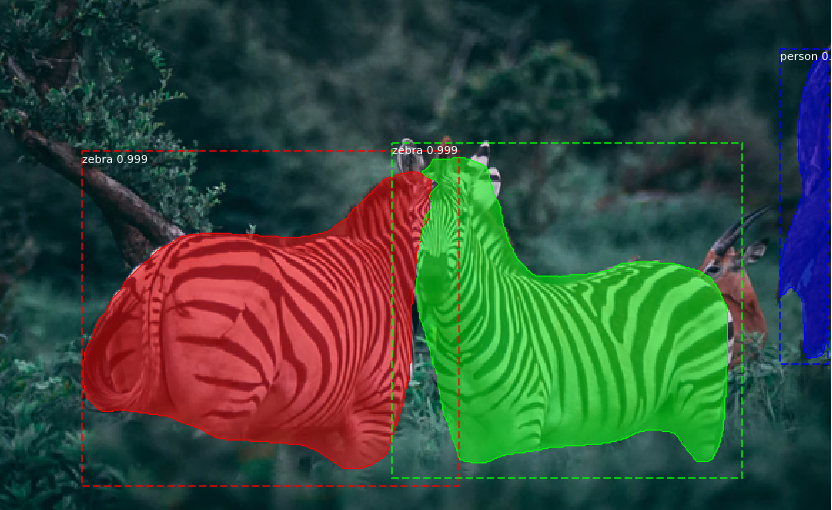# Evaluating performance of an object detection model

Source: Deep Learning on Medium# Evaluating performance of an object detection model

## What is mAP ? How to evaluate the performance of an object detection model?

In this article you will figure out how to use mAP to evaluate the performance of an object detection model .What is mAP? How to calculate mAP along with 11-point interpolation?

We use machine learning and deep learning to solve regression or classification problem.

We used Root Mean Square(RMS) or Mean Average Percentage Error(MAPE) etc. to evaluate the performance of a regression model.

Classification models are evaluated using Accuracy, Precision, Recall or an F1- Score.

Is object detection, a classification or a regression problem?

Multiple deep learning algorithms exists for object detection like RCNN’s : Fast RCNN, Faster RCNN, YOLO, Mask RCNN etc.

Objective of an object detection models is to

• Classification :Identify if an object is present in the image and the class of the object
• Localization : Predict the co-ordinates of the bounding box around the object when an object is present in the image. Here we compare the co-ordinates of ground truth and predicted bounding boxes

We need to evaluate performance of both classification as well as localization of using bounding boxes in the image

How do we measure the performance of object detection model?

For object detection we use the concept of Intersection over Union (IoU). IoU computes intersection over the union of the two bounding boxes; the bounding box for the ground truth and the predicted bounding box

An IoU of 1 implies that predicted and the ground-truth bounding boxes perfectly overlap.

You can set a threshold value for the IoU to determine if the object detection is valid or not not.

Let’s say you set IoU to 0.5, in that case

• if IoU ≥0.5, classify the object detection as True Positive(TP)
• if Iou <0.5, then it is a wrong detection and classify it as False Positive(FP)
• When a ground truth is present in the image and model failed to detect the object, classify it as False Negative(FN).
• True Negative (TN): TN is every part of the image where we did not predict an object. This metrics is not useful for object detection, hence we ignore TN.

Set IoU threshold value to 0.5 or greater. It can be set to 0.5, 0.75. 0.9 or 0.95 etc.

Use Precision and Recall as the metrics to evaluate the performance. Precision and Recall are calculated using true positives(TP), false positives(FP) and false negatives(FN).